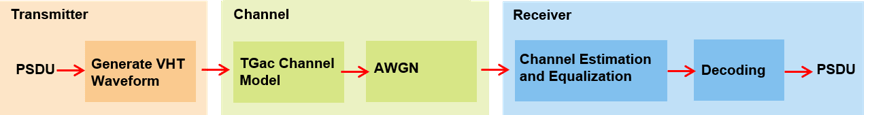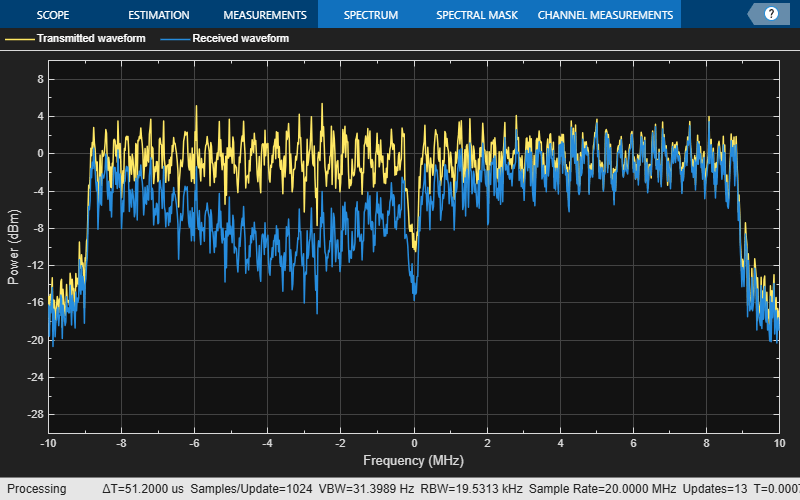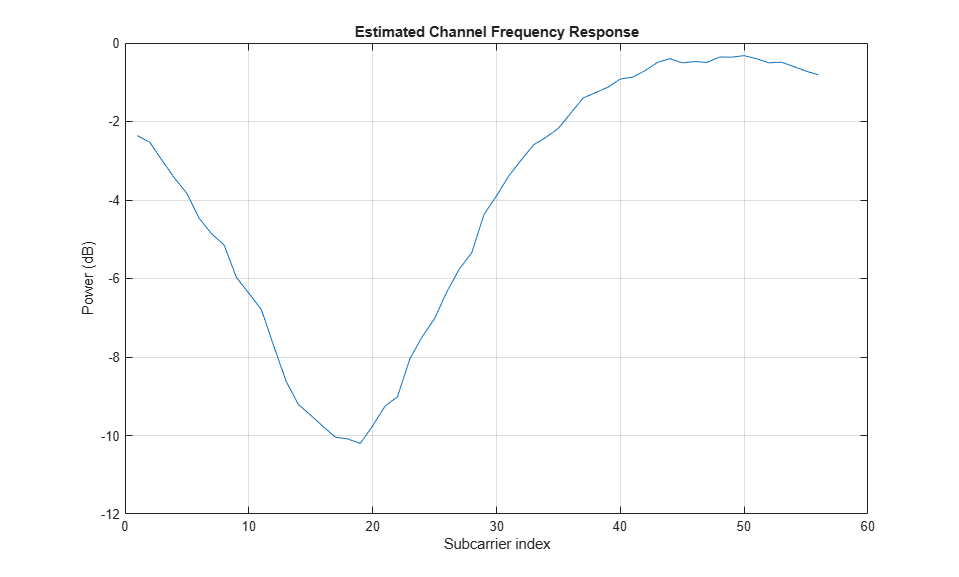This example shows how to create a basic WLAN link model using WLAN Toolbox™. An IEEE® 802.11ac™ [ 1 ] VHT packet is created, passed through a TGac channel. The received signal is equalized and decoded in order to recover the transmitted bits.

### Introduction

This example shows how a simple transmitter-channel-receiver simulation may be created using functions from WLAN Toolbox. A VHT transmit and receive link is implemented as shown in the figure below. A VHT packet is transmitted through a TGac channel, demodulated and the equalized symbols are recovered. The equalized symbols are decoded to recover the transmitted bits.### Waveform Generation

An 802.11ac VHT transmission is simulated in this example. The transmit parameters for the VHT format of the 802.11™ standard are configured using a VHT configuration object. The `wlanVHTConfig` creates a VHT configuration object. In this example the object is configured for a 20 MHz channel bandwidth, MCS 5 and single transmit antenna.

```% Create a format configuration object for a SISO VHT transmission cfgVHT = wlanVHTConfig; cfgVHT.NumTransmitAntennas = 1; % Transmit antennas cfgVHT.NumSpaceTimeStreams = 1; % Space-time streams cfgVHT.APEPLength = 4096; % APEP length in bytes cfgVHT.MCS = 5; % Single spatial stream, 64-QAM cfgVHT.ChannelBandwidth = 'CBW20'; % Transmitted signal bandwidth Rs = wlanSampleRate(cfgVHT); % Sampling rate ```

A single VHT packet is generated consisting of training, signal and data fields:

• Non-HT Short Training Field (L-STF)

• Non-HT Long Training Field (L-LTF)

• Non-HT Signal (L-SIG) field

• VHT Signal A (VHT-SIG-A) field

• VHT Short Training Field (VHT-STF)

• VHT Long Training Field (VHT-LTF)

• VHT Signal B (VHT-SIG-B) field

• Data field

These fields are generated separately using functions from WLAN Toolbox and are concatenated to produce a VHT transmit packet.

The first field in the PPDU is the L-STF and is used for the start of packet detection and automatic gain control (AGC) setting. It is also used for initial frequency offset estimation and coarse timing synchronization. The `wlanLSTF` function generates the L-STF field in the time-domain using some of the parameters included in configuration object `cfgVHT`.

```lstf = wlanLSTF(cfgVHT); ```

The L-LTF is used for fine time synchronization, channel estimation and fine frequency offset estimation. The docid:wlan_ref#buz04s0 wlanLLTF> function generates the L-LTF in the time-domain.

```lltf = wlanLLTF(cfgVHT); ```

The L-SIG field carries packet configuration such as data rate, modulation and code rate for non-HT format. The `wlanLSIG` function generates the L-SIG field in the time-domain.

```lsig = wlanLSIG(cfgVHT); ```

The figure below shows the L-STF, L-LTF and L-SIG fields. These fields are common to the VHT, HT-Mixed and non-HT OFDM transmission formats.

```nonHTfield = [lstf;lltf;lsig]; % Combine the non-HT preamble fields ```The VHT specific signal and training fields are generated after the non-HT preamble fields. The purpose of the VHT-SIG-A field is to provide information to allow the receiver to decode the data payload. The VHT-SIG-A is composed of two symbols VHT-SIG-A1 and VHT-SIG-A2. The `wlanVHTSIGA` function generates the VHT-SIG-A field in the time-domain.

```vhtsiga = wlanVHTSIGA(cfgVHT); ```

The purpose of the VHT-STF is to improve the gain control estimation in a MIMO transmission and help the receiver detect the repeating pattern similar to the L-STF field. The `wlanVHTSTF` function generates the VHT-STF field in the time-domain.

```vhtstf = wlanVHTSTF(cfgVHT); ```

The VHT-LTF provides a mean for the receiver to estimate the channel between the transmitter and the receiver. Depending on the number of space time streams, it consists of 1,2,4,6 or 8 VHT-LTF symbols. The `wlanVHTLTF` function generates the VHT-LTF in the time-domain.

```vhtltf = wlanVHTLTF(cfgVHT); ```

The VHT-SIG-B field is used to set the data rate and the length of the data field payload of the transmitted packet. The `wlanVHTSIGB` function generates the VHT-SIG-B field in the time-domain.

```vhtsigb = wlanVHTSIGB(cfgVHT); ```

Construct the preamble with the generated signal and training fields for the VHT format.

```preamble = [lstf;lltf;lsig;vhtsiga;vhtstf;vhtltf;vhtsigb]; ```

The `wlanVHTData` function generates the time-domain VHT data field. The VHT format configuration `cfgVHT` specifies the parameters for generating the data field from the PSDU bits. The `cfgVHT.PSDULength` property gives the number of bytes to be transmitted in the VHT data field. This property is used to generate the random PSDU bits `txPSDU`.

```rng(0) % Initialize the random number generator txPSDU = randi([0 1],cfgVHT.PSDULength*8,1); % Generate PSDU data in bits data = wlanVHTData(txPSDU,cfgVHT); % A VHT waveform is constructed by prepending the non-HT and VHT % preamble fields with data txWaveform = [preamble;data]; % Transmit VHT PPDU ```

Alternatively the waveform for a given format configuration can also be generated using a single function call `wlanWaveformGenerator` function. This function can produce one or more VHT packets. By default OFDM windowing is applied to the generated waveform. For more information on OFDM windowing, see the reference page for the `wlanWaveformGenerator` function.

### Channel Impairments

This section simulates the effects of over-the-air transmission. The transmitted signal is impaired by the channel and AWGN. The level of the AWGN is given in dBs. In this example the TGac channel model [ 2 ] is used with delay profile Model-B. For this delay profile when the distance between transmitter and receiver is greater than or equal to 5 meters, the model is in Non-Line-of-Sight (N-LOS) configuration. This is described further in the help for `wlanTGacChannel`.

```% Parameterize the channel tgacChannel = wlanTGacChannel; tgacChannel.DelayProfile = 'Model-B'; tgacChannel.NumTransmitAntennas = cfgVHT.NumTransmitAntennas; tgacChannel.NumReceiveAntennas = 1; tgacChannel.LargeScaleFadingEffect = 'None'; tgacChannel.ChannelBandwidth = 'CBW20'; tgacChannel.TransmitReceiveDistance = 5; tgacChannel.SampleRate = Rs; tgacChannel.RandomStream = 'mt19937ar with seed'; tgacChannel.Seed = 10; % Pass signal through the channel. Append zeroes to compensate for channel % filter delay txWaveform = [txWaveform;zeros(10,1)]; chanOut = tgacChannel(txWaveform); snr = 40; % In dBs rxWaveform = awgn(chanOut,snr,0); % Display the spectrum of the transmitted and received signals. The % received signal spectrum is affected by the channel spectrumAnalyzer = dsp.SpectrumAnalyzer('SampleRate',Rs, ... 'AveragingMethod','Exponential','ForgettingFactor',0.99, ... 'YLimits',[-30 10],'ShowLegend',true, ... 'ChannelNames',{'Transmitted waveform','Received waveform'}); spectrumAnalyzer([txWaveform rxWaveform]); ```### Channel Estimation and Equalization

In this section the time-domain VHT-LTF is extracted from the received waveform. The waveform is assumed to be synchronized to the start of the packet by taking the channel filter delay into account. The VHT-LTF is demodulated and is used to estimate the channel. The received signal is then equalized using the channel estimate obtained from the VHT-LTF.

In this example the received signal is synchronized to the start of the packet by compensating for a known channel filter delay. For more information on how to automatically detect and synchronize to the received signal see the following examples:

```chInfo = info(tgacChannel); % Get characteristic information % Channel filter delay, measured in samples chDelay = chInfo.ChannelFilterDelay; rxWaveform = rxWaveform(chDelay+1:end,:); ```

After synchronization the receiver has to extract the relevant fields from the received packet. The `wlanFieldIndices` function is used to return the start and end time-domain sample indices of all fields relative to the first sample in a packet. These indices are used to extract the required fields for further processing.

```indField = wlanFieldIndices(cfgVHT); ```

An estimate of the noise power after OFDM demodulation is required to perform MMSE equalization on the received OFDM symbols. In this example the noise power in the VHT fields is estimated using the demodulated L-LTF symbols.The L-LTF is extracted from the received waveform and is demodulated using the `wlanLLTFDemodulate` function.

```indLLTF = indField.LLTF(1):indField.LLTF(2); demodLLTF = wlanLLTFDemodulate(rxWaveform(indLLTF),cfgVHT); % Estimate noise power in VHT fields nVar = helperNoiseEstimate(demodLLTF,cfgVHT.ChannelBandwidth,cfgVHT.NumSpaceTimeStreams); ```

To extract the VHT-LTF from the received signal the start and end indices are used to generate a vector of indices.

```indVHTLTF = indField.VHTLTF(1):indField.VHTLTF(2); ```

The VHT-LTF is used to estimate the channel between all space-time streams and receive antennas. The VHT-LTF is extracted from the received waveform and is demodulated using the `wlanVHTLTFDemodulate` function.

```demodVHTLTF = wlanVHTLTFDemodulate(rxWaveform(indVHTLTF,:),cfgVHT); ```

The channel estimate includes the effect of the applied spatial mapping and cyclic shifts at the transmitter for a multi antenna configuration. The `wlanVHTLTFChannelEstimate` function returns the estimated channel between all space-time streams and receive antennas.

```chanEstVHTLTF = wlanVHTLTFChannelEstimate(demodVHTLTF,cfgVHT); ```

The transmit signal encounters a deep fade as shown in the channel frequency response in the figure below. The effect of channel fades can also be seen in the spectrum plot shown previously.

```figure plot(20*log10(abs(chanEstVHTLTF))); grid on; title('Estimated Channel Response'); xlabel('Subcarrier index'); ylabel('Power (dB)'); ```To extract the data field from the received signal the start and end indices for the data field are used to generate a vector of indices.

```indData = indField.VHTData(1):indField.VHTData(2); % Recover the bits and equalized symbols in the VHT Data field using the % channel estimates from VHT-LTF [rxPSDU,~,eqSym] = wlanVHTDataRecover(rxWaveform(indData,:),chanEstVHTLTF,nVar,cfgVHT); % Compare transmit and receive PSDU bits numErr = biterr(txPSDU,rxPSDU); ```

The following plot shows the constellation of the equalized symbols at the output of the `wlanVHTDataRecover` function compared against the reference constellation. Increasing the channel noise should begin to spread the distinct constellation points.

```% Plot equalized symbols constellationDiagram = comm.ConstellationDiagram; constellationDiagram.ReferenceConstellation = wlanReferenceSymbols(cfgVHT); % Compare received and reference constellation constellationDiagram(reshape(eqSym,[],1)); constellationDiagram.Title = 'Equalized Data Symbols'; ```### Appendix

This example uses this helper function.

### Selected Bibliography

1. IEEE Std 802.11ac™-2013 IEEE Standard for Information technology - Telecommunications and information exchange between systems - Local and metropolitan area networks - Specific requirements - Part 11: Wireless LAN Medium Access Control (MAC) and Physical Layer (PHY) Specifications - Amendment 4: Enhancements for Very High Throughput for Operation in Bands below 6 GHz.

2. Breit, G., H. Sampath, S. Vermani, et al. TGac Channel Model Addendum. Version 12. IEEE 802.11-09/0308r12, March 2010.

## SupportGet trial now